# Description¶

In this section you will get to know the key notions that will be used through the course.

## Data science¶

Data science - is a field that uses scientific methods, processes, algorithms and systems to extract knowledge and insights from structured and unstructured data. Data science is a field that combines statistics, data analysis and machine learning to understand and analyse the data. Nowadays data science is one of the most demanding professions in sphere of IT, as the contemporary world is a data driven one. The main duties of every data scientists include : analysis of data, it’s preprocessing, cleaning and transformation and finally extracting sense from it using statistical and machine learning techniques along with presentation of performed results to non technical people. As the core of data science is statistics and mathematics, the related part of the course is focused on teaching how to apply the techniques of highlighted spheres to solve real world problems.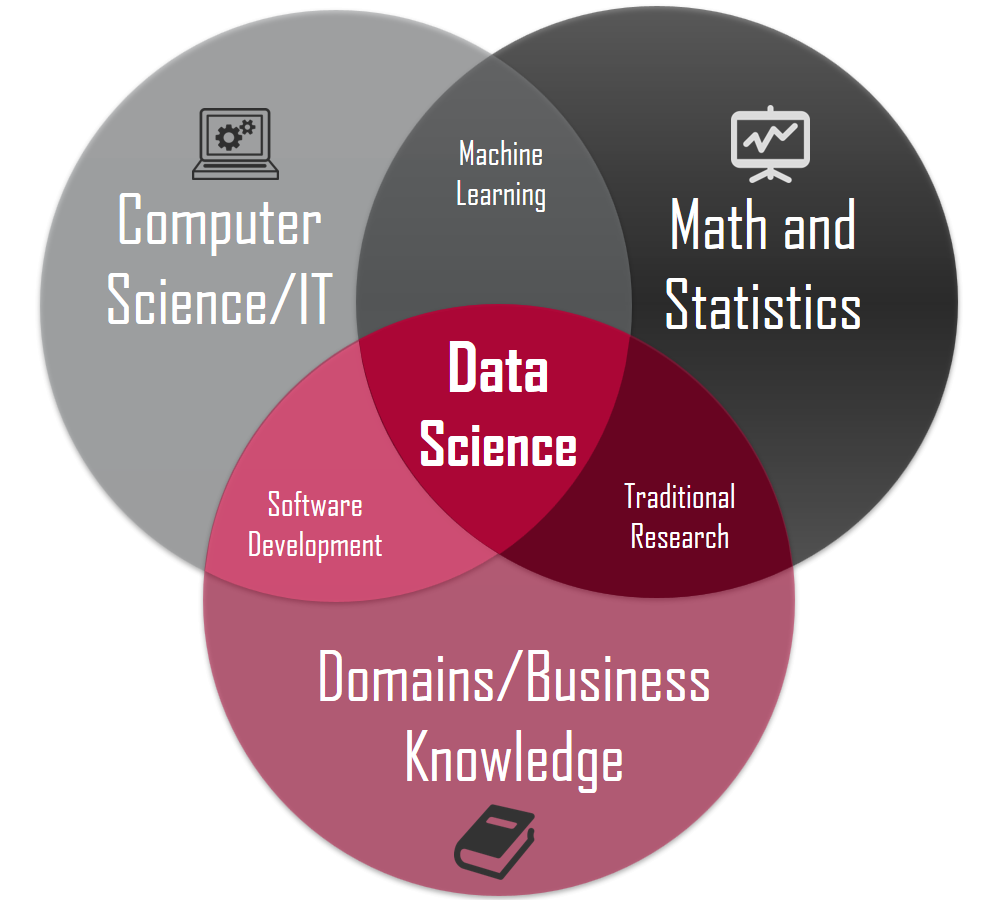## Machine learning¶

Machine learning - is a branch of AI (Artificial Intelligence) in programming the main goal of which is to make the machine “learn” how to solve the tasks which can’t be programmed explicitly. Nowadays, Machine learning is a really hot topic, as the applications of this sphere extended broadly. For instance let’s consider the contemporary smartphone : such functions as voice assistant, fingerprint and face verification, handwritten recognition are included by default, and all of the highlighted functions are machine learning driven. Actually, to understand the main difference between machine learning and ordinary programming we can expose the following example :

Let’s say we need to make a program that should predict the price of the house depending on the number of its rooms (the example is simplified). We can try to solve this program without using any machine learning techniques, just by writing some if else rules. But as the data grows it will become harder and harder to code all the rules to obtain an expected results. A much smarter solution is to train a machine learning algorithm that will find the dependency between price and number of rooms and then create the rules itself (the current example is related to supervised learning)
The picture describing this main difference is pinned below.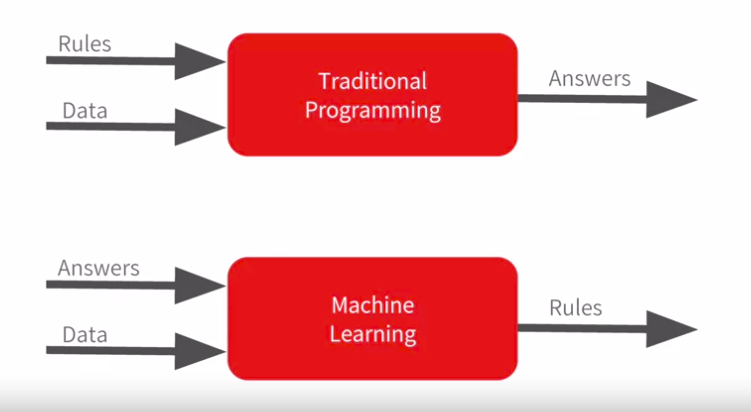The are three main types of machine learning :

• Supervised learning

• Unsupervised learning

• Reinforcement learning

Note

We won’t consider the Reinforcement learning in this course, but you can find additional information about it here. Instead we will mainly focus on “classical machine learning”.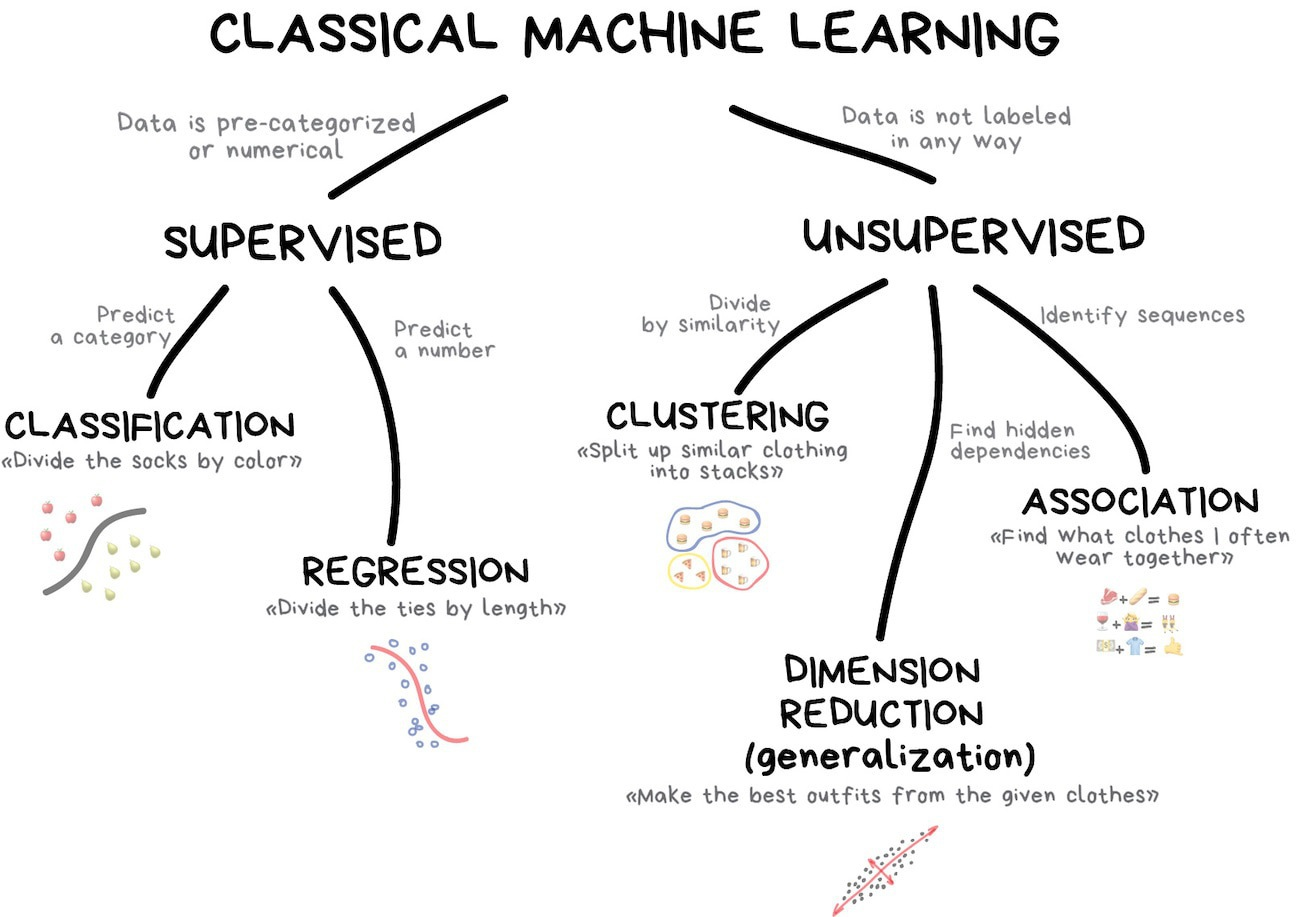Supervised learning - is a type of machine learning, when given a data/features (by notation X) and corresponding answers/labels (by notation Y) an algorithm learns a complex function to map data/features to answers/labels. There are lots of useful application concerning supervised learning, for instance : image classification, fraud detection, object recognition, face verification, weather forecast, etc. The supervised learning is divided into two types of problems : regression and classification.

In classification problem the answer (sometimes called the target, we will use this name further) is a categorical label/class and the task of the algorithm (sometimes called the classifier or the model) is to classify the sample/object depending on the features of relevant data. For better understanding, let’s say you encountered the following problem : you have pictures of cats and dogs and you should determine whether the picture contains dog or cat. That’s a routine task of image classification.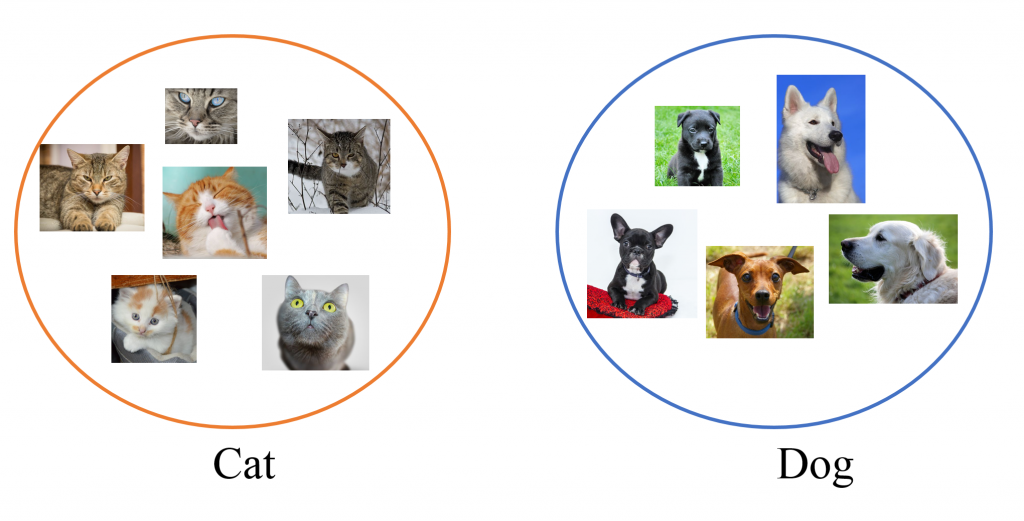On contrary the problem of regression exists, in which the target is a discrete continuous number and the task of a model is to learn the dependencies between features and the target to output the value that is the nearest to the target one. Simple example of regression is the task of predicting houses’ prices depending on the number of rooms/size of the house. Instead of classifying houses into different groups, what we really want is to predict its exact price, that is actually a continuous number.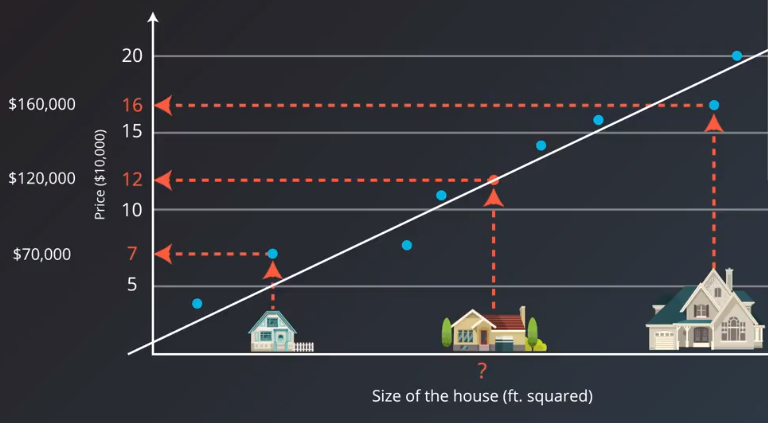Unsupervised learning - is a type of learning when algorithm is given only data/features without any answers/labels. The purpose of unsupervised learning algorithms is to find the similarities between data samples and based on this similarities perform some actions. The unsupervised learning is divided into three types of problems : clustering , dimension reduction , association.

Note

We will focus only on clustering as the other algorithms are out of the scope in this course, but we encourage you to visit this page to get more information.

In clustering problem the goal of the algorithm is to cluster the data into different groups based on the similarities between samples. To understand the gist of clustering let’s define the following problem : you need to make a system that identifies spam messages and sends them to spam folder. What you can do is gather the information like words from the messages and than break these information into two different groups, after that you can determine yourself which group contains spam and which - not.

To understand the difference between supervised and unsupervised learning let’s consider the following pictures which shows the difference between classification (supervised learning) and clustering (unsupervised learning) :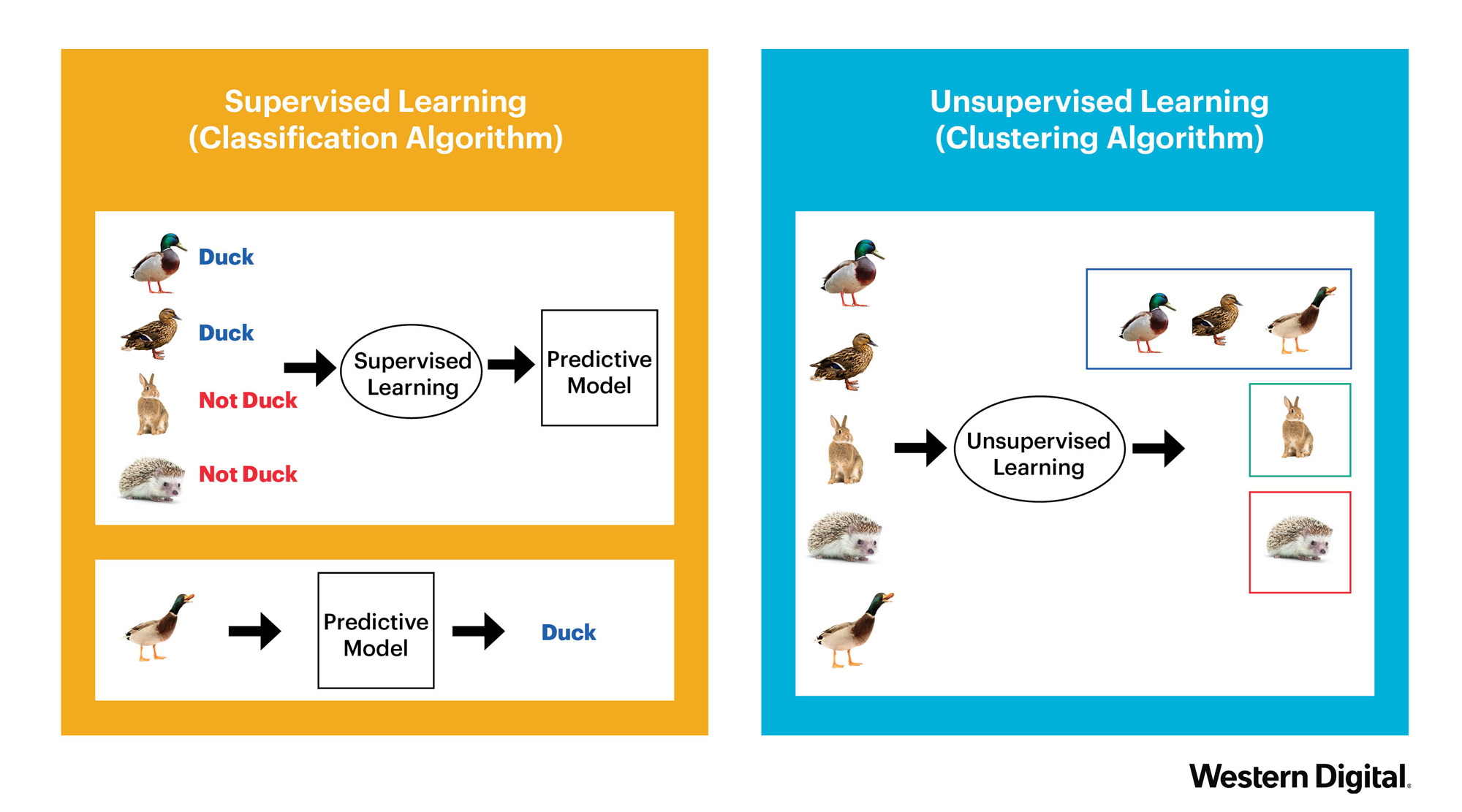You will have a chance to work with both supervised and unsupervised learning techniques and dig deeper into core concepts of machine learning further in this course.

## Python¶

Why use Python for machine learning and data science? The answer is pretty obvious, because it’s much simpler, much faster and finally much more efficient to do this heavy job using the exposed programming language. Python scientific packages such as scipy, numpy, pandas and others allow conducting complex mathematics computations and statistics calculus in few lines of code giving analysts and researchers a possibility to easily make analysis and developing new algorithms. What is more, Python is usually used in production solutions, thus you can easily refactor your draft code for (let’s say) processing of the data and then scale it up to production system.

Despite the fact that in this course you won’t write the production ready code, you will get to know how to use Python for basic analysis and machine learning that will give you the mandatory skills to continue learning and developing in data science area. Finally, to persuade you in the fact that Python is the language you should really use, let’s look at the chart showing the popularity of languages for the current year :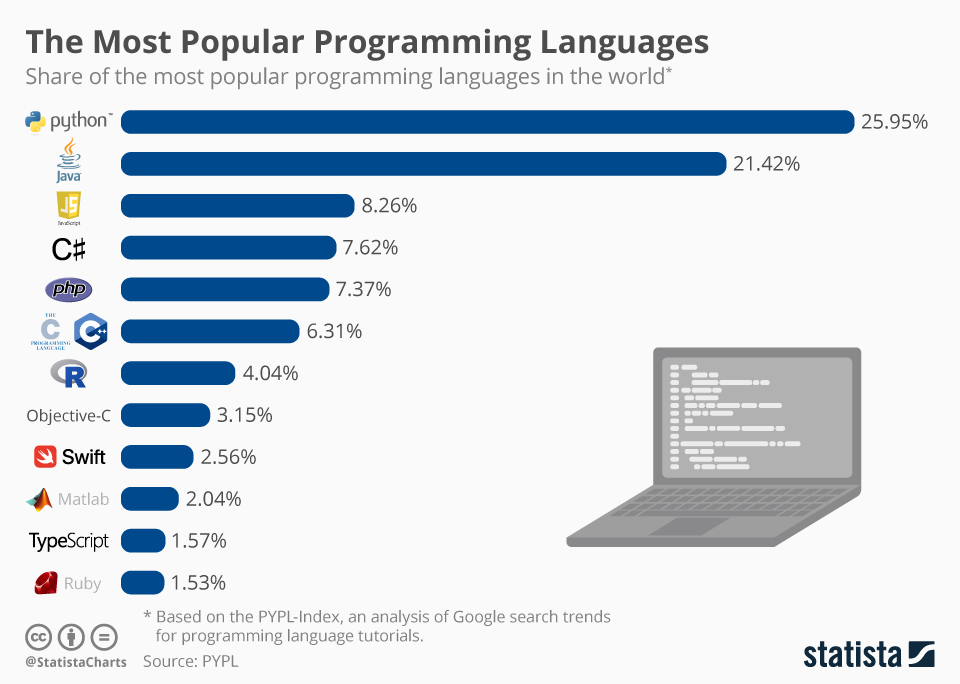Based on the diagram shown above, Python is the most popular language at the moment, just analytics, nothing personal.